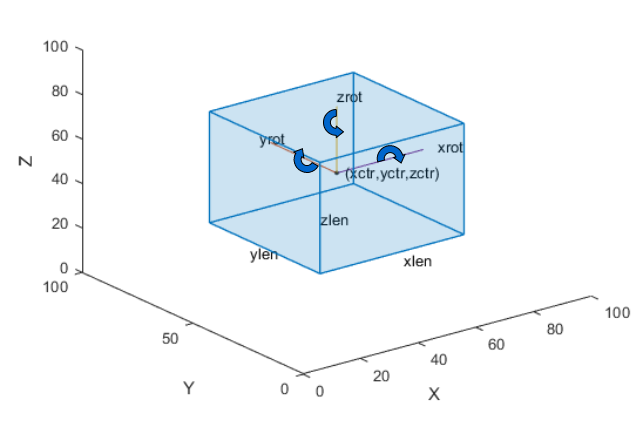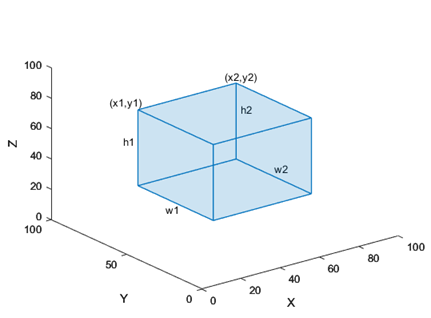# run

Class: vision.labeler.AutomationAlgorithm
Package: vision.labeler

Run label automation on every frame in interval

## Syntax

``autoLabels = run(algObj,frame)``

## Description

The `run` method computes the automated labels for a single frame by executing the automation algorithm. During automation, the labeling apps run this method in a loop to compute the automated labels for each frame in the selection being automated.

Clients of `AutomationAlgorithm` must implement this method.

````autoLabels = run(algObj,frame)` processes a single frame, `frame`, using the `algObj` automation algorithm, and returns the automated labels, `autoLabels`.```

## Input Arguments

expand all

Automation algorithm, specified as a `vision.labeler.AutomationAlgorithm` object.

Frame whose labels are being computed, specified as a numeric matrix for `Image` signals or a `pointCloud` object for `PointCloud` signals.

## Output Arguments

expand all

Labels produced by the automation algorithm, returned as a categorical matrix, structure array, or table.

For algorithms that automate pixel labeling, implement the `run` method to return `autoLabels` as a `categorical` label matrix, where each category represents a pixel label. For more information, see How Labeler Apps Store Exported Pixel Labels.

For algorithms that automate nonpixel labels, implement the `run` method to return a structure array. Each structure in the array contains the labels of a specific name and type. The method combines labels of the same name and type into a single structure in the array.

This table describes the columns of the `autoLabels` table or fields of each `autoLabels` structure.

Field NameDescription
`Type`

`labelType` enumeration that contains the type of the label. Valid label types are:

• `labelType.Rectangle`

• `labelType.Cuboid` (Ground Truth Labeler app only)

• `labelType.ProjectedCuboid`

• `labelType.Line`

• `labelType.Scene`

`Name`Character vector containing the name of the label.
`Position `

Position of labels of the specified `Name` and `Type`. The format of `Position` depends on the label type.

Label Type`Position` Format
`Rectangle` — Rectangular region of interest (ROI) labels

`M`-by-4 numeric matrix of the form `[x, y, w, h]`, where:

• `M` is the number of labels in the frame.

• `x` and `y` specify the upper-left corner of the rectangle.

• `w` specifies the width of the rectangle, which is its length along the x-axis.

• `h` specifies the height of the rectangle, which is its length along the y-axis.

`Cuboid` — Cuboid ROI labels

`M`-by-9 numeric matrix with rows of the form `[xctr, yctr, zctr, xlen, ylen, zlen, xrot, yrot, zrot]`, where:

• `M` is the number of labels in the frame.

• `xctr`, `yctr`, and `zctr` specify the center of the cuboid.

• `xlen`, `ylen`, and `zlen` specify the length of the cuboid along the x-axis, y-axis, and z-axis, respectively, before rotation has been applied.

• `xrot`, `yrot`, and `zrot` specify the rotation angles for the cuboid along the x-axis, y-axis, and z-axis, respectively. These angles are clockwise-positive when looking in the forward direction of their corresponding axes.

The figure shows how these values determine the position of a cuboid.`ProjectedCuboid` — Cuboid ROI labels

`M`-by-8 vector of the form ```[x1, y1, w1, h1, x2, y2, w2, h2]```, where:

• `M` is the number of labels in the frame.

• `x1, y1` specifies the x,y coordinates for the upper-left location of the front-face of the projected cuboid

• `w1` specifies the width for the front-face of the projected cuboid.

• `h1` specifies the height for the front-face of the projected cuboid.

• `x2, y2` specifies the x,y coordinates for the upper-left location of the back-face of the projected cuboid.

• `w2` specifies the width for the back-face of the projected cuboid.

• `h2` specifies the height for the back-face of the projected cuboid.

The figure shows how these values determine the position of a cuboid.`Line` — Polyline ROI labels

`M`-by-1 vector of cell arrays, where `M` is the number of labels in the frame. Each cell array contains an `N`-by-2 numeric matrix of the form `[x1 y1; x2 y2; ... ; xN yN]` for `N` points in the polyline.

`Scene` — Scene labels

Logical value of `1` if the algorithm determines that the label is present in the frame and `0` otherwise.

`Attributes` (optional)

Structure array that contains one structure for each attribute in the label. If the label definition does not contain attributes, then the `autoLabels` output does not include this field.

For each structure in the `Attributes` structure array, the name of that structure is the name of the corresponding attribute. The value of the structure is the value of the corresponding attribute.

To view a sample `autoLabels` structure array, enter this code at the MATLAB® command prompt.

```autoLabels(1).Name = 'Car'; autoLabels(1).Type = labelType.Rectangle; autoLabels(1).Position = [20 20 50 50]; autoLabels(2).Name = 'Truck'; autoLabels(2).Type = labelType.Rectangle; autoLabels(2).Position = [70 70 50 50]; ```

Alternatively, for nonpixel labels, you can use the `run` method to return `autoLabels` as a table. The table rows are equivalent to the structures in a structure array. The table columns are equivalent to the structure fields. This table is equivalent to the sample `autoLabels` structure array previously specified.

``` Name Type Position ____________ _________ ____________ 'Car' Rectangle [1x4 double] 'Truck' Rectangle [1x4 double]```